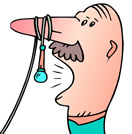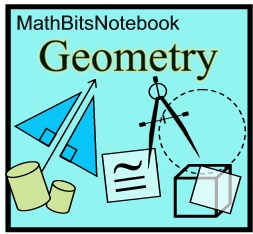Practice with Translations Terms of Use    Contact Person: Donna Roberts1.
A translation function is defined by the rule
(x, y) → (x + 2, y - 5). Which choice will be the image of the point (3,6) under this translation?
Choose:
 (3,11) (5,11) (5,1) (6,1)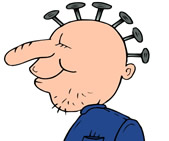2.
Which translation mapping is depicted in the graph at the right?

Choose:
 (x, y) → (x + 6, y - 3) (x, y) → (x - 3, y + 6) (x, y) → (x - 6, y + 3) (x, y) → (x + 3, y - 6)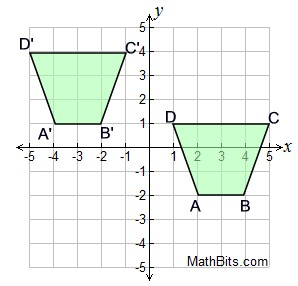3.
A graphic design uses two congruent rectangles as color blocks to hold the artist's signature. Rectangle S'P'A'T' is the translation of rectangle SPAT, as shown in the table at the right.
a) What are the coordinates of A ?
b) What are the coordinates of P' ?
c) Write the translation function that was used in this design.
 Rectangle SPAT Rectangle S'P'A'T' S (-3,2) S' (-1,1) P (1, 2) P' A A' (3,-2) T (-3,-1) T' (-1,-2)

4.
The translation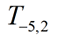will move point (-4,-7) to the point _____.
Choose:
 (-1,-9) (1,-5) (-9,-9) (-9,-5)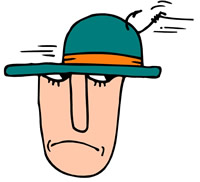5.
Regarding the graph at the right:
a) Which point is the image of point A under (x, y) → (x - 5, y - 2)?
Choose:
 B C D E

b) Which point is the image of point C under (x, y) → (x + 4, y + 1)?
Choose:
 A B D E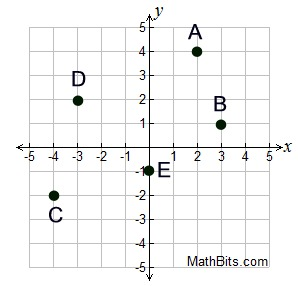c)
Which point is the image of point B under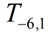?
Choose:
 A C D E

6.
A translation maps the origin to the point (-2,4). What is the image of point (-5,3) under this same translation?
Choose:
 (-7, 7) (-3, -1) (-3, 7) (-7, 1)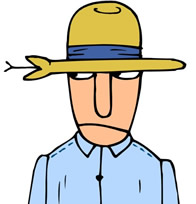7.
Given ΔPQR with P(-2,3), Q(3,7), and R(7,2).
A translation of
(x, y) → (x + 4, y - 3) is applied to ΔPQR.
a) What are the coordinates of point P after the translation?
Choose:
 (2,4) (6,2) (2,0) (6,4)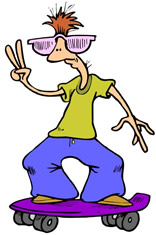b)
What is the distance from point P to point P' ?

Choose:
 3 units 4 units 5 units 6 units

8.
The vertices of rectangle MATH are M(-4,2),
A
(-4,5), T(3,5) and H(3,2).
a) Find the area of rectangle MATH in square units.

Choose:
 15 square units 21 square units 35 square units 36 square units

b)
Translate the rectangle 3 units to the right and 4 units down. Find the area of rectangle M'A'T'H' in square units.

Choose:
 15 square units 21 square units 35 square units 36 square units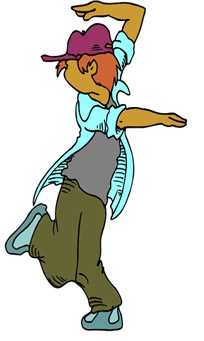9.
The diagram at the right shows 16 dots from a sheet of dot paper, where the dots are equally spaced. A translation maps dot B to dot K.
Under this translation to which dot will dot E be mapped?

Choose:
 C J O N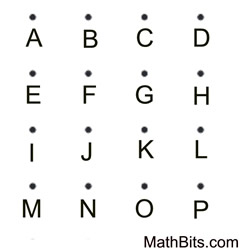10 Explain why there can be no "fixed" points under a translation, other than the translation T0,0.